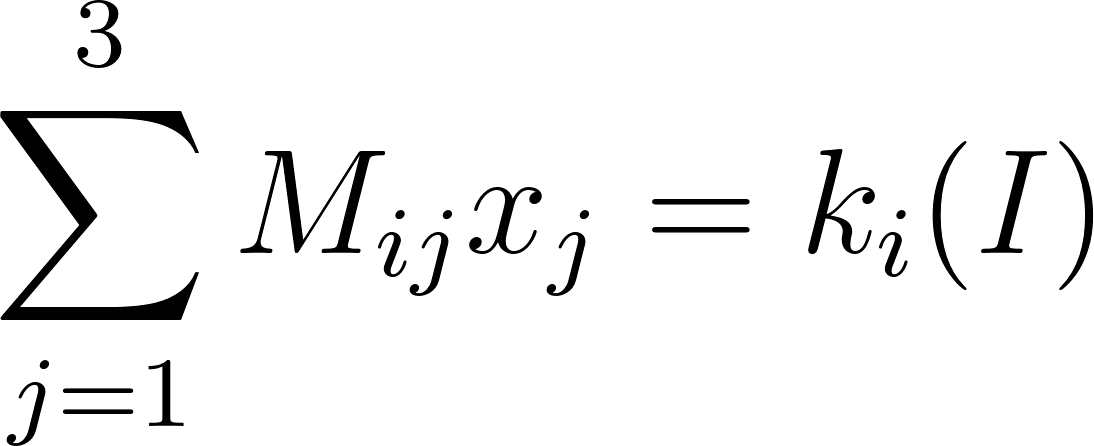### Solution Found!# The first equation in (2.6) written out in detail is M11x1 + M12x2 + M13x3 = k1. Write

Chapter 3, Problem 1

(choose chapter or problem)

Get Unlimited Answers! Check out our subscriptions
QUESTION:

The first equation in (2.6) written out in detail is M11x1 + M12x2 + M13x3 = k1. Write out the other two equations in the same way and then substitute x1, x2, x3 = x, y, z and the values of Mij and ki from (2.4) and (2.5) to verify that (2.6) is really (2.3).

QUESTION:

The first equation in (2.6) written out in detail is M11x1 + M12x2 + M13x3 = k1. Write out the other two equations in the same way and then substitute x1, x2, x3 = x, y, z and the values of Mij and ki from (2.4) and (2.5) to verify that (2.6) is really (2.3).

Step 1 of 3

We know thatSo the summation can be written as